Measurement of Excess & Partial Mol Volume of Ethanol Water Mixtures Lab Report

THE EFFECT OF COMPOSITION ON SEVERAL PHYSICAL PROPERTIES OF BINARY MIXTURES OF COMMON LIQUIDS

# Objective

The effect of the composition of solutions on their physical properties has been an important focus of both experimental and theoretical investigations in physical chemistry for many years. This multipart project involves the determination of the effect of composition on the partial molar volume, viscosity and surface tension for a binary mixture. Appropriate selection of components for the mixtures insures interesting variations in these properties with composition. Comparison of the whole range of properties invites a consistent interpretation of the observed composition dependence of these properties at the molecular level.

The entire project can be completed in two or three 3-hour laboratory periods with students working in groups. An additional period is allocated to complete workup of the data and preparation of a formal report. Coming early in the first semester of the course, the workup of the data provides a good introduction to (or review of) the use of spreadsheet software and computer graphing of data. A powder-point presentation will be made for comparison of results among the groups and to provide opportunities for students to give oral reports on their investigations.

# Preparation of Mixture Solution

1. Two binary mixture solutions will be studied in this project and each group will be assigned to study one mixture. A series of such binary solutions of 100 ml need to be prepared at the beginning of the project. Please follow the instruction below to make corresponding solutions.
 Mixture Component 1 Component 2 Group 1 Ethanol Water A, C 2 Ethanol Acetone B, D

1. Prepare a series of ethanol-water mixture (for mixture 2, different liquids should be used) according to the following steps:
1. Weigh a clean and dry 100-mL volumetric flask on the analytical balance.
2. Use a graduated cylinder to obtain 20 mL of ethanol and add it to the flask. Record the actual mass. (You may use stopper if reading is not stable due to evaporation.)
3. Fill the volumetric flask with deionized water until the liquid level reaches 100-ml mark. Shake the mixture gently. Reweigh the flask and record the actual mass. You have the first ethanol-water mixture. Using the data collected here, you should be able to calculate the mole fraction of ethanol in the mixture and thus the mole fraction of water accordingly.
4. Repeat step (a) through step (c) with a combination of 40 mL ethanol / 60 mL water, 60 mL ethanol / 40 mL water, 80 mL ethanol / 20 mL water, and 95 mL ethanol / 5 mL water. Record the masses you actually added in each mixing. Now you have four more ethanol-water mixtures.
5. Save each of mixtures in a clean plastic bottle and tighten the cap to avoid evaporation. Label all five bottles to make sure you are able to identify their compositions. You are going to measure the physical properties of these mixtures in the next several experiments.

# Safety Practice

• You must wear your safety glasses.
• Avoid inhalation of gas vapor.

EXPERIMENT 6

MEASUREMENT OF EXCESS VOLUME AND PARTIAL MOLAR VOLUME OF

ETHANOL-WATER MIXTURES

# I. Obiective

The objective of this experiment is to illustrate the partial molar quantities in a mixture by the determination of the excess volume in a series of ethanol-water solutions of known composition.

# 2. Introduction

Every bartender knows well that mixing 50 ml of water with 50 ml of ethanol does not give an alcohol drink of 100 ml; the difference is called the excess volume. This excess volume can be expressed in terms of the mole fraction of either ethanol or water, and the magnitude of the excess volume provides a

measurement of non-ideal mixing of water and ethanol molecules. The existence of the excess volume indicates that molar volumes are non-additive. Instead, thermodynamics dictates that only the partial molar properties are additive.

The volume of a solution or a liquid mixture is dependent on its temperature (T) and pressure (p) and the amount of each component (m, n2 used to form the mixture. For a binary system (A and B) held at constant temperature and pressure, we can write:

dV = VAdnA + VBdng (Eq. 1)

where VA and VB are the partial molar volumes of substance A and B in the mixture, which can be defined as follows:where the subscript n’ signifies that the amount of all other substances present are constant.

Equation 1 implies that when the composition of the mixture is changed by the addition of dnn of A and dng of B, then the change in the total volume of the mixture can be calculated by Equation 1. Provided that the relative composition is held constant as the amounts of A and B are increased, we can obtain the final volume by integrating Equation 1:

V = VAt1A + Vang (Eq. 3)

where V is the actual/real volume of a solution and can calculated from the density of mixture.

If V* is the ideal volume of a binary solution based on the molar volumes of the two components at constant temperature and pressure.

(Eq. 4)

where and are the molar volumes of component A and B as pure substance.

The excess volume VE can then calculated by

## VE -V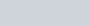+14m) – + v;ng)

We have discussed some details concerning partial molar volumes in Physical Chemistry lectures. Let us use an ethanol-water mixture as an example. At 20 o c the molar volumes of water and ethanol are:v; o = 18.0 mL mor

V• H —58.0 mL mol -I

If the molar volumes of ethanol and water do not change upon mixing, we can predict the volume of an aqueous ethanol solution when 1.0 mol of water is mixing with 1.0 mol of ethanol as:

V* +1xVEt0H= rnL mol-I +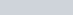rnL mor

= 76.0 ml

However the actual volumes – the partial molar volumes – of the substances in the mixture do change. At a mole fraction of XEtOH = 0.5, the partial molar volumes of the substances in the mixture are:

VHO = 16.9 mL mol-I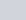H = 57.4 mL mol -I

Thus for our example solution, we have a total volume of: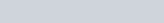H = mL mol-I +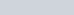mL mol -I

= 74.3 rnL

In other words, the excessive volume of the ethanol-water mixture is

VE = 74.3 mL mol -I — 76.0 mL mol-I = -1.7 mL mol-ICan Accommodate EtOH’s Tail

Stlllcture Of “Water”

The volume contracts when these two substances are mixed at this composition. This situation should not be unexpected. Liquid water has a fairly open structure so that the voids in its structure are “filled” with the non-polar hydrocarbon portion of the ethanol molecules upon mixing. This packing of the molecules in the mixtures results in the contraction of the volume from simply adding the volumes of the pure substances together.

In this experiment we will prepare a series of ethanol-water mixtures and then determine the densities p of these mixtures using a calibrated volumetric flask (A.K.A. Pycnometer). The excess volume V E for a 1 mol ethanol-water mixture is related to the density p at a given temperature by:

(MH 0 +MEt0H&t0H)

# — (VHIOXH O + )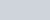where MH o and M H are the molar mass of water and ethanol, respectively. v; o and V• H are the molar volumes of pure water and ethanol at a given temperature, respectively. The actual values can be calculated after we have determined the densities of pure water and pure ethanol from this experiment.

3. Safety Practice

You must wear your safety glasses.

## 4. Experimental Procedure

We will be making the required density measurements using a 25-mL volumetric flask. Each mass measurement has to be as accurate as possible. Please wait until the reading is stable and record the whole number you read off from the balance.

(I) Weigh a dry and clean 25 ml- volumetric flask.

1. Fill the flask to the mark with deionized water until the liquid level is near to the top of the ground surface. Put the stopper in the flask in order to drive our all the air and any excess water. Work the stopper gently into the flask, so that it is firmly seated in position. Wipe any water from the outside of the flask with a paper towel and soak up all excess water from around the top of the stopper.
2. Reweigh the flask, which should be completely dry on the outside and full of water and absolutely no air. Given the density of water at current room temperature (Please record it) and the mass of the water, you should be able to determine the volume of the flask very precisely.
3. Empty the flask and dry it. Repeat step 2 and 3 using pure ethanol and the four ethanol-water mixtures you prepared earlier. Record data accordingly.
4. Calculate the density of all mixtures first and then calculate the excess volumes. Include appropriate error estimates. List all of your results in a Table.
5. Make a plot of excess volume VE as a function of the mole fraction of ethanol.
6. From the plot above, determine the partial molar volumes of ethanol and water at each concentrations (Please do your research to find out how to do this)
7. Make a graph, showing how the real molar volume of mixture (V), the partial molar volumes of ethanol (V Et0H ) , as well as the partial molar volumes of water ( VH20 ) change with the mole fraction of ethanol.

## 5. Questions and Further Thoughts

(I) Is your plot of excess volume VE as a function of the mole fraction of ethanol expected?

1. At which mole fraction of ethanol, the deviation from ideal solution is largest? Explain this from the molecular perspective.
2. How do your results compare to those in literature?

## 6. Reference

(1) P. W. Atkins, “Physical Chemistry”, 9th edition, Chapter 5 — Simple Mixtures